# Lab 10.2 - Latent Profile Analysis

## Structural Equation Modeling - Instructor: Karen Nylund-Gibson

### June 07, 2020

University of California, Santa Barbara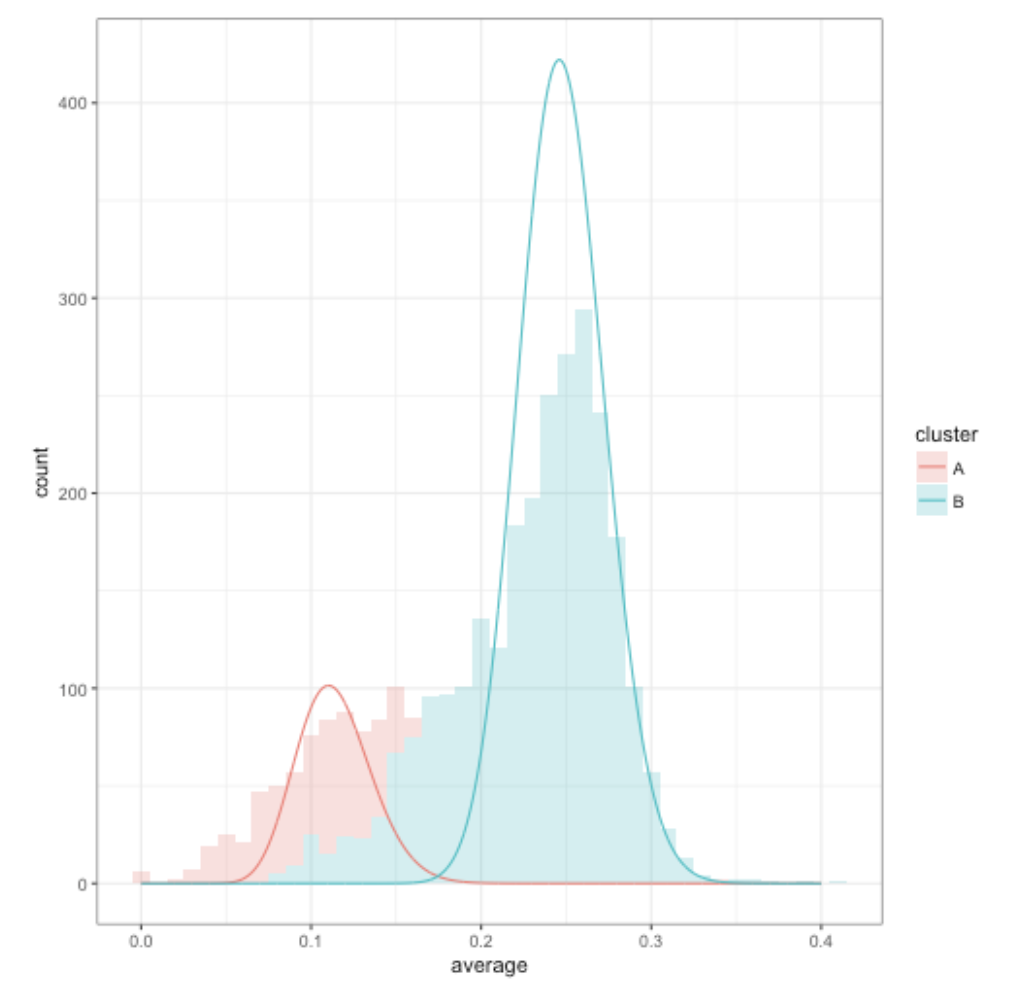Figure. Gaussian mixture models. Data simulated from a 2-class model.

# Lab preparation

## Creating a version-controlled R-Project with Github

On the Github repository webpage:

1. fork your own branch of the lab repository
2. copy the repository web URL address from the clone or download menu

Within R-Studio:

1. click “NEW PROJECT”
2. choose option Version Control
3. choose option Git
4. paste the repository web URL path copied from the clone or download menu on Github page
5. choose location of the R-Project

## Data source:

1. The first example closely follows the vignette used to demonstrate the tidyLPA package (Rosenberg, 2019): $$\color{blue}{\text{See detailed documentation of this model here}}$$

This model utilizes the PISA data collected in the U.S. in 2015. To learn more about this data $$\color{blue}{\text{see here}}$$.

To access the 2015 US PISA data in R use the following code: a. devtools::install_github("jrosen48/pisaUSA15") b. library(pisaUSA15) c. open_codebook()

1. The second examples utilizes 4 test score measures from the public-use dataset, The Longitudinal Survey of American Youth (LSAY): $$\color{blue}{\text{See documentation here}}$$

library(naniar)
library(tidyverse)
library(haven)
library(glue)
library(MplusAutomation)
library(rhdf5)
library(here)
library(janitor)
library(gt)
library(tidyLPA)

pisa <- pisaUSA15

# Latent Profile Analysis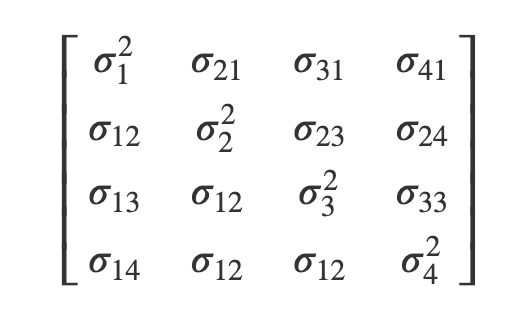Figure. Picture adapted from tutorial (Rosenberg, 2019).

• model 1: Equal variances, and covariances fixed to 0 (Class-invariant / Diagonal)
• model 2: Free variances and covariances fixed to 0 (Class-varying / Diagonal)
• model 3: Equal variances and equal covariances (Class-invariant / Non-Diagonal)
• model 4: Free variances, and equal covariances
• model 5: Equal variances, and free covariances
• model 6: Free variances and free covariances (Class Varying / Non-Diagonal)

## Example 1: PISA dataset from the tidyLPA package

Enumerate using estimate_profiles():

• Estimate models with classes $$K = 1:3$$ using Mplus
• Model has 4 continuous indicators
• Default variance-covariance sprecifications is model 1
• In the followind example model 1 and model 6 are compared
• Add line scale() %>% to center indicator means
lpa_models <- pisa[1:500,] %>%
estimate_profiles(1:3,
package = "MplusAutomation",
ANALYSIS = "starts = 100, 20;",
variances = c("equal", "varying"),
covariances = c("zero", "varying"))

get_fit(lpa_models)

Plot 3-class model

Note: single imputation is used in this example as plot_profiles() requires complete cases

pisa[1:200,] %>%
single_imputation() %>%
estimate_profiles(3, package = "MplusAutomation") %>%
plot_profiles(sd=FALSE)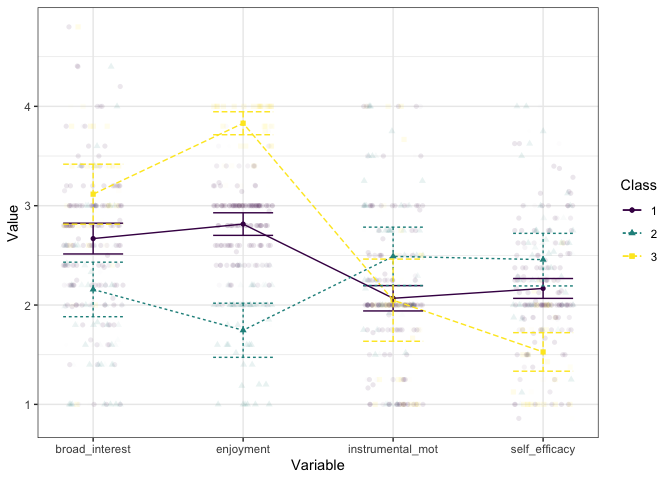Plot densities for classes k = 1:4

pisa[1:500, c("broad_interest","enjoyment")] %>%
single_imputation() %>%
estimate_profiles(1:4, package = "MplusAutomation") %>%
plot_density()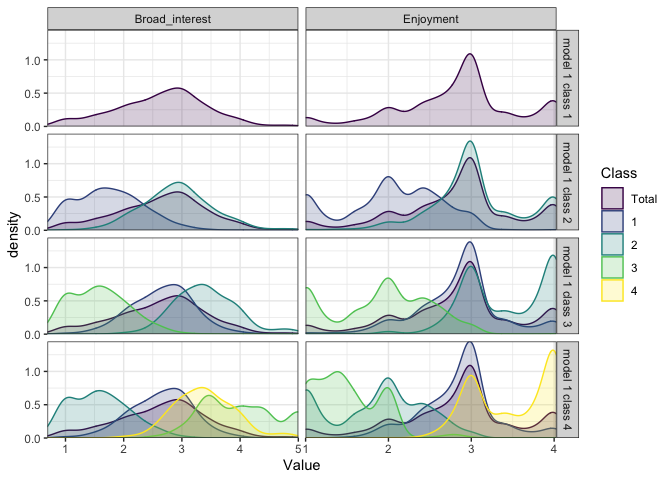## Example 2: Math, Science, Physics, and Biology measures (LSAY).

lsay_data <- read_csv(here("data", "lsay_lab10.2_lpa.csv"))

A. Run a quick enumeration (default variance-covariance specification - model 1)

lpa_k14  <- lapply(1:4, function(k) {
lpa_enum  <- mplusObject(

TITLE = glue("Class {k}A"),

VARIABLE = glue(
"usevar = mth_scor-bio_scor;
classes = c({k}); "),

ANALYSIS =
"estimator = mlr;
type = mixture;
starts = 200 50;
processors = 10;",

OUTPUT = "sampstat residual tech11 tech14;",

PLOT =
"type = plot3;
series = mth_scor-bio_scor(*);",

usevariables = colnames(lsay_data),
rdata = lsay_data)

lpa_enum_fit <- mplusModeler(lpa_enum,
dataout=glue(here("enum_lpa", "c_lpaA_lsay_Lab10.dat")),
modelout=glue(here("enum_lpa", "c{k}_lpaA_lsay_Lab10.inp")) ,
check=TRUE, run = TRUE, hashfilename = FALSE)
})

Plot $$1:4$$ class model profiles

lsay_data[1:500,5:8] %>%
single_imputation() %>%
estimate_profiles(1:4, package = "MplusAutomation") %>%
plot_profiles(sd=FALSE)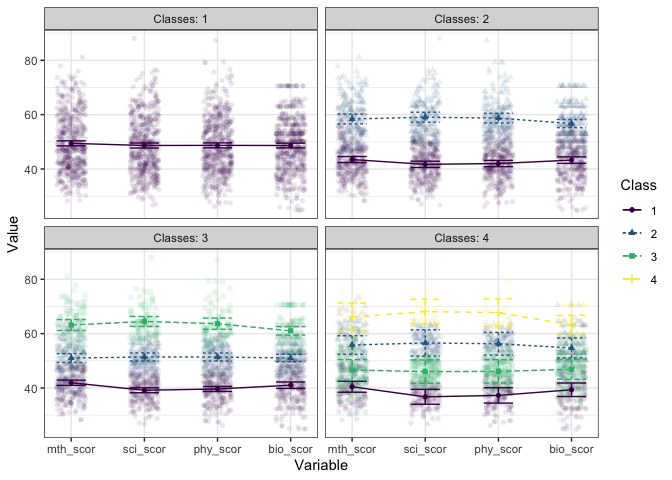Figure. Here we see ordered solutions.

This result might be aniticipated given that the correlation between test scores ranges from moderate to high (r = .57 - .95).

Compare model fit.

all_output <- readModels(here("enum_lpa"), quiet = TRUE)

enum_extract <- LatexSummaryTable(all_output,                                             #
keepCols=c("Title","Parameters", "LL", "BIC",                             #
"aBIC", "BLRT_PValue", "T11_VLMR_PValue"),                     #
sortBy = "Title")                                                         #

gt(enum_extract)

## Which variance-covariance specification is appropriate?

In this tutorial we consider the following three models: A. equal variances and covariances fixed to zero (default) B. free variances and covariances fixed to zero (Model 2) C. free variances and free covariance for Physics and Science test scores (Model 6)